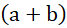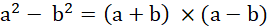# RD Sharma Class 9 and Chapter Factorization Of Polynomial Maths Solutions

## Maths Class 9 and Chapter Factorization Of Polynomial RD Sharma SolutionsFilter
Filters :
CLASSES
•
•
•
•
•
•
•
CHAPTERS
•
• 15 More

# RD Sharma Class 9 Maths Chapter 6 Factorization of Polynomials

The previous chapters of class 9 RD Sharma’s books discussed on the basics of algebra such as the algebraic expressions, algebraic identities and factorisation of algebraic expressions. Thus, the students following the textbook would, by now, have a vague idea about the terms such as identities, factorisation and the polynomials. The RD Sharma Class 9 Maths Chapter 6, again talks about the factorization of polynomials, in-depth and through this, the students would gain a thorough understanding of concepts such as the ‘degree of an expression’, terms of a polynomial, co-efficient of a polynomial, factor theorem, reminder theorem, etc.

Before getting into this chapter, it is important to understand that it is vital to refer to the right study material. The right study material helps the students in sailing through the process of learning a subject as difficult as Maths in a smooth way. And here comes the textbook of RD Sharma, which has served as a great support to the students studying the CBSE syllabus for many years now. RD Sharma’s textbooks are widely preferred as they provide the latest syllabus content. The best thing about these textbooks are the concepts explained in a deep but concise manner. The students are backed with many solved example problems as well as the end number of exercise-wise practise problems. Practising these problems are sufficient not just to ace the examinations but also to ace the top entrance exams in India like the IIT JEE. It is important for the aspirants to concentrate on learning the fundamental concepts of Maths right now in a proper way, and the RD Sharma books, serve as the best choice of learning materials that satisfy this purpose.

It is a well-known fact that practising problems from the RD Sharma books for Maths discard a lot of worries pertaining to maths among the students. Nevertheless, mere solving the textbook problems would not always help, but referring to a good solution guide alongside solving the textbook problems would take the learning process to a whole new level. Now, this is where our online tutorial platform, doubtnut.com, comes in and proves to be helpful for the students by providing video solutions. All the solutions from the RD Sharma books can be found on doubtnut.com and these solution videos can be accessed from anywhere and through any internet enabled device. RD Sharma Class 9 Solutions Chapter 6 Factorization of Polynomials found on doubtnut.com covers all the important concepts and identities that are vital in order to know the factorization of polynomials. Let us now look at what exactly constitutes the RD Sharma chapter 6, Factorization of Polynomials.

Factorization of Polynomials Class 9 Maths RD Sharma Solutions

It is known that the integers can be represented in the form of multiples of their smallest terms and each of these multiples is nothing, but a factor. For example, the number 15 can be represented in the form of multiples as, 3*5 = 15. Now, the numbers 3 and 5 form the factors of the number 15 and this process is called factorization. This process of factorization can be applied to algebraic expressions as well and this whole concept has been covered in the previous unit of the RD Sharma book. The previous unit saw a few methods that would aid in the factorization of algebraic expressions. These methods revolved around factorizing the monomial, binomial or trinomial expressions in simple ways. This unit sees using the factorization of polynomials and popular methods to factorize the polynomials.

A polynomial, just like the numbers can be written in the forms of its factors. A lot of times, factorisation of polynomials commonly involves methods such as the grouping of the common terms. For an example, the expression 4x2 – x = 0, can be written as x (4x – 1) = 0. Further, we it can be written as x = 0 and x = ¼. Another method of factorizing the polynomials is the method of splitting the middle terms, mainly in binomial and trinomial equations and this was called the ‘factor method of factorization’. And finally, the process of factorization using algebraic identities was seen. Solving the polynomial equations and quadratic equations would involve using a combination of various methods of factorizations. In many cases, factorization of algebraic equations involves using the standard algebraic identities. Recollecting the commonly used algebraic identities,

a+b2 = a2 + 2ab + b2

a+b2 = a2 + 2ab + b2

a2- b2=a+b ×(a-b)(a + b)= a3 + b3 + 3ab (a + b)

(a - b)= a3 - b3 - 3ab (a - b)

a3 + b3 = (a + b) (a2 + b2 – ab)

a3 – b3 = (a – b) (a2 + b2 – ab)

(x + y +z)2=x2 +y2 + z2+2xy+2yz+2x

So, in this unit, apart from these previously covered methods of factorization, deals with further high-level concepts such as the Remainder theorem and factorization using the Factor theorem to solve the problems. Apart from these, the concepts of coefficient and the degree of the polynomial are introduced here and one can find a lot of problems from these concepts in the textbook exercise section. The related terms such as linear polynomial, cubic polynomial, zero polynomial, zeroes of a polynomial, etc. One can spot the problems related to these concepts in the practise section of the textbook. It is also important to identify if an expression is a polynomial in one variable or not. These are the new concepts that are covered under this unit in RD Sharma’s book and it is very important to learn these before going any further through the learning of the Algebra.

Our online tutorial platform, Doubtnut, provides all that essential for the students in sailing through the process of learning the Algebra part of mathematics according to the CBSE syllabus, smoothly.  The solutions for the exercise problems of the chapter factorization of polynomials is worked out exercise-wise making it really easy for the students to follow.

Topics and Subtopics of RD Sharma Class 9 Maths Chapter 6 Factorization of Polynomials

Having seen a brief about all that covered in this unit from RD Sharma’s textbook, it is now time to look at the topics and subtopics covered under RD Sharma Class 9 Maths Chapter 6 Factorization of Polynomials. Before going into exploring any unit, it is important to be aware of the topics and subtopics covered in order to better plan the study timetable in a systematic way. One can see that the video solutions on doubtnut.com are based on these topics and subtopics of RD Sharma Class 9 Maths Chapter 6 Factorization of Polynomials:

### Polynomials in one variable

Here the concepts of zero polynomial, terms of a polynomial, finding the coefficients of a polynomial, constant polynomial, and the terms such as monomial, binomial, trinomial, linear polynomial, cubic polynomial, and quadratic polynomial are introduced here.

### Zeroes of a polynomial

Finding out a number ‘c’ that satisfies the condition p(c) = 0 of the equation p(x) is called finding the zeroes of a polynomial. Here, the other related concepts such as finding the roots of the polynomials are introduced.

### Remainder theorem

The theorem that is used to solve the problems that revolve around finding the reminders when divided by their factors and also the problems that involve checking if a term makes the factor of expression or not.

### Factor theorem

This topic covers the process of factorization of polynomials by using the factor theorem. One can find exercise problems based on this concept, too.

All these concepts are best covered in the form of solutions on the doubtnut.com and this will come as the need of the hour especially during the time of examinations. The learners don’t have to pile upon their doubts that could arise during the learning process of factorization of the polynomials. Students can get them easily cleared by the team of talented subject matter experts at any time by just posting the picture of the doubts on our online portal, Doubtnut.com.  The methodically solved problems presented by an expert teacher through the pre-recorded videos ensure the students don’t have to fret anymore during the examination time. The videos can be played, paused, replayed end number of times until the learners get more and more confident with the concepts. Doubtnut has now garnered the attention of thousands of students across India and they find both the app and the online portal very handy, especially during self-study.

All the video solutions are presented in a step-by-step way so that even a naïve learner can easily understand the nuances involved when cracking the complex concepts. This has made our platform stand out amongst the premium online tutorial platforms in India!